﻿ How To Group, Concatenate & Merge Data in Pandas – BMC Software | Blogs
Machine Learning & Big Data Blog

# How To Group, Concatenate & Merge Data in PandasIn this tutorial, we show how to group, concatenate, and merge Pandas DataFrames. (New to Pandas? Start with our Pandas introduction or create a Pandas dataframe from a dictionary.)

These operations are very much similar to SQL operations on a row and column database. Pandas, after all, is a row and column in-memory data structure. If you’re a SQL programmer, you’ll already be familiar with all of this. The only complexity here is that you can join by columns in addition to rows.

Pandas uses the function concatenation—concat(), aka concat. But it’s easier to understand if you think of these are inner joins (intersection) and outer joins (union) of sets, which is how I refer to it below.

(This tutorial is part of our Pandas Guide. Use the right-hand menu to navigate.)

## Concatenation (Outer join)

Think of concatenation like an outer join. The result is the same.

Suppose we have dataframes A and B with common elements among the indexes and columns.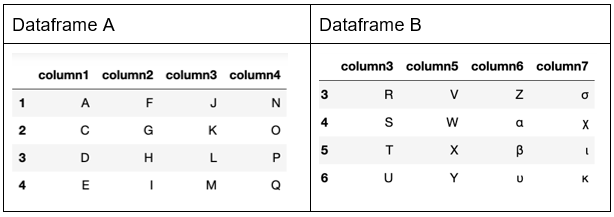Now concatenate. It’s not an append. (There is an append() function for that.) This concat() operation creates a superset of both sets a and b but combines the common rows. It’s not an inner join, either, since it lists all rows even those for which there is no common index.

Notice the missing values NaN. This is where there are no corresponding dataframe indexes in Dataframe B with the index in Dataframe A.

For example, index 3 is in both dataframes. So, Pandas copies the 4 columns from the first dataframe and the 4 columns from the second dataframe to the newly constructed dataframe. Similarly, index 5 is in Dataframe B but not Dataframe A for columns 1,2, 3. So those columns are marked as missing (NaN).

```a = pd.DataFrame({'column1': ['A', 'C', 'D', 'E'],

'column2': ['F', 'G', 'H', 'I'],

'column3': ['J', 'K', 'L', 'M'],

'column4': ['N', 'O', 'P', 'Q']},

index=[1,2,3,4])

b = pd.DataFrame({'column3': ['R', 'S', 'T', 'U'],

'column5': ['V', 'W', 'X', 'Y'],

'column6': ['Z', 'α', 'β', 'υ'],

'column7': ['σ', 'χ', 'ι', 'κ']},

index=[3,4,5,6])

result = pd.concat([a, b], axis=1)
```

Results in: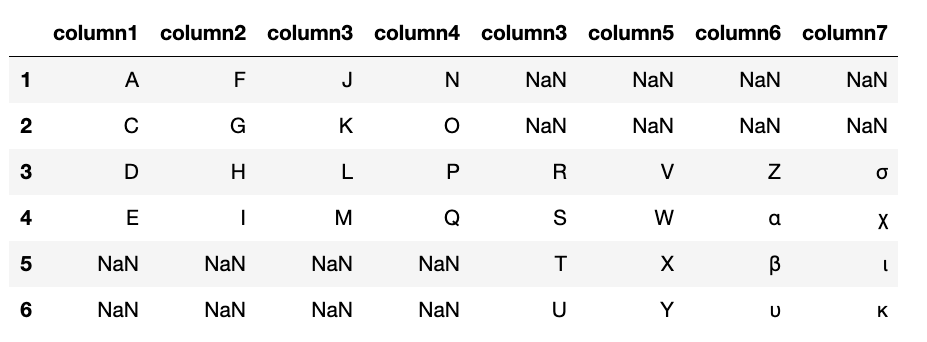## Outer join

Here we do an outer join, which, in terms of sets, means the union of two sets. So, all rows are added. An outer join here does not create the intersection of common indexes. But still, for those for which the column does not exist in the set of all columns the value is NaN. That has to be the case since not all columns exist for all rows. So, you have to list all of them but mark some of them as empty.

`result = pd.concat([a, b], join='outer')`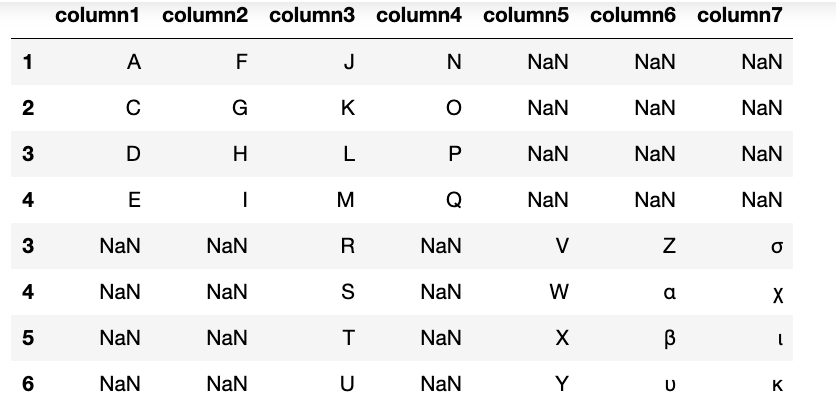## Inner join along the 0 axis (Row)

We can do an inner join by index or column. An inner join finds the intersection of two sets.

Let’s join along the 0 axis (row). Only indices 3 and 4 are in both dataframes. So, an inner join takes all columns from only those two rows.

`result = pd.concat([a, b], axis=1,join='inner')`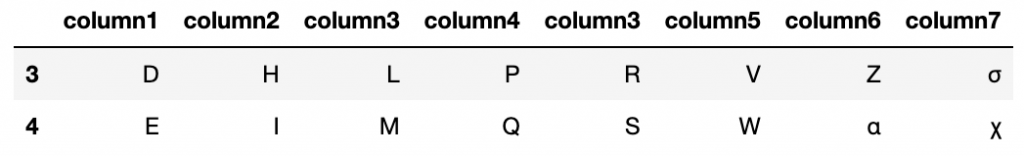## Inner join along the 1 axis (Column)

Column3 is the only column common to both dataframe. So, we concatenate all the rows from A with the rows in B and select only the common column, i.e., an inner join along the column axis.

`result = pd.concat([a, b], axis=0,join='inner')`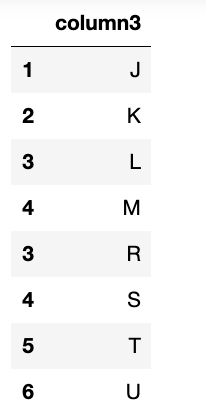## Merge

A merge is like an inner join, except we tell it what column to merge on.

Here, make the first column name (i.e., key value in the dictionary) some common name “key”. Then we merge on that.

```a = pd.DataFrame({'key': ['A', 'C', 'D', 'E'],

'column2': ['F', 'G', 'H', 'I'],

'column3': ['J', 'K', 'L', 'M'],

'column4': ['N', 'O', 'P', 'Q']},

index=[1,2,3,4])

b = pd.DataFrame({'key': ['C', 'D', 'T', 'U'],

'column5': ['V', 'W', 'X', 'Y'],

'column6': ['Z', 'α', 'β', 'υ'],

'column7': ['σ', 'χ', 'ι', 'κ']},

index=[3,4,5,6])

result=pd.merge(a, b, on='key')```

The resulting dataframe is the one which has a common key value from the array key=[].You see that only C and D are common to both data frames.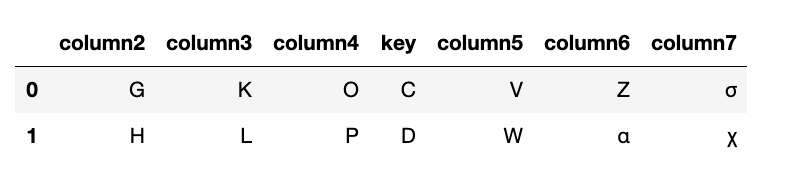## Append

This simply appends one dataframe onto another. Here is a Series, which is a DataFrame with only one column. The result is all rows from Dataframe A added to Dataframe B to create Dataframe C.

```import pandas as pd

a=pd.DataFrame([1,2,3])

b=pd.DataFrame([4,5,6])

c=a.append(b)

c```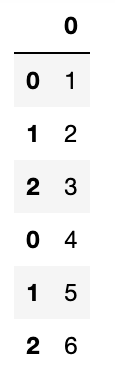## GroupBy

Here is another operation familiar to SQL programmers.

The GroupBy operation is on a single dataframe. We group the values from the column named key and sum them.

```a=pd.DataFrame({"key": ["a", "b", "c","a", "b", "c"] ,

"values": [1,2,3,1,2,3]})

b=a.groupby("key").sum()

b```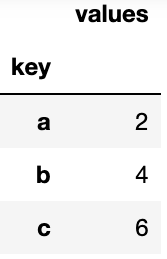This e-book teaches machine learning in the simplest way possible. This book is for managers, programmers, directors – and anyone else who wants to learn machine learning. We start with very basic stats and algebra and build upon that.

These postings are my own and do not necessarily represent BMC's position, strategies, or opinion.

See an error or have a suggestion? Please let us know by emailing blogs@bmc.com.

### BMC Bring the A-Game

From core to cloud to edge, BMC delivers the software and services that enable nearly 10,000 global customers, including 84% of the Forbes Global 100, to thrive in their ongoing evolution to an Autonomous Digital Enterprise.